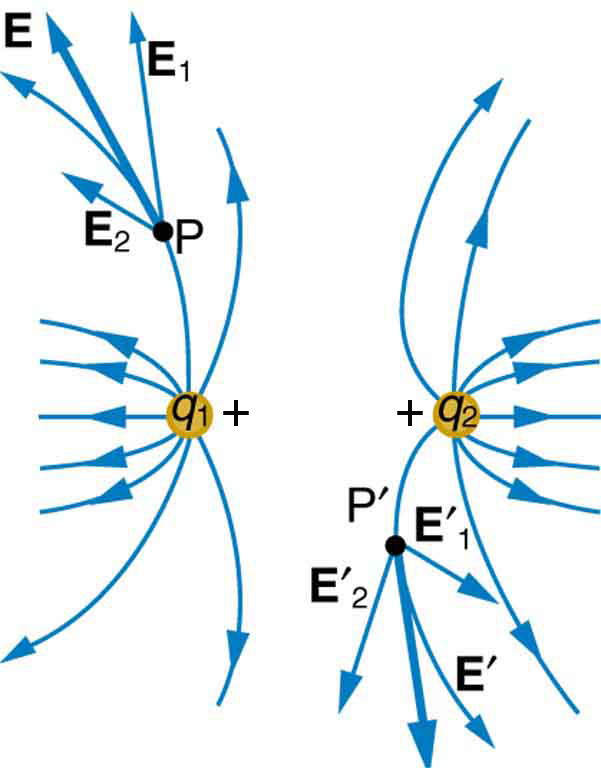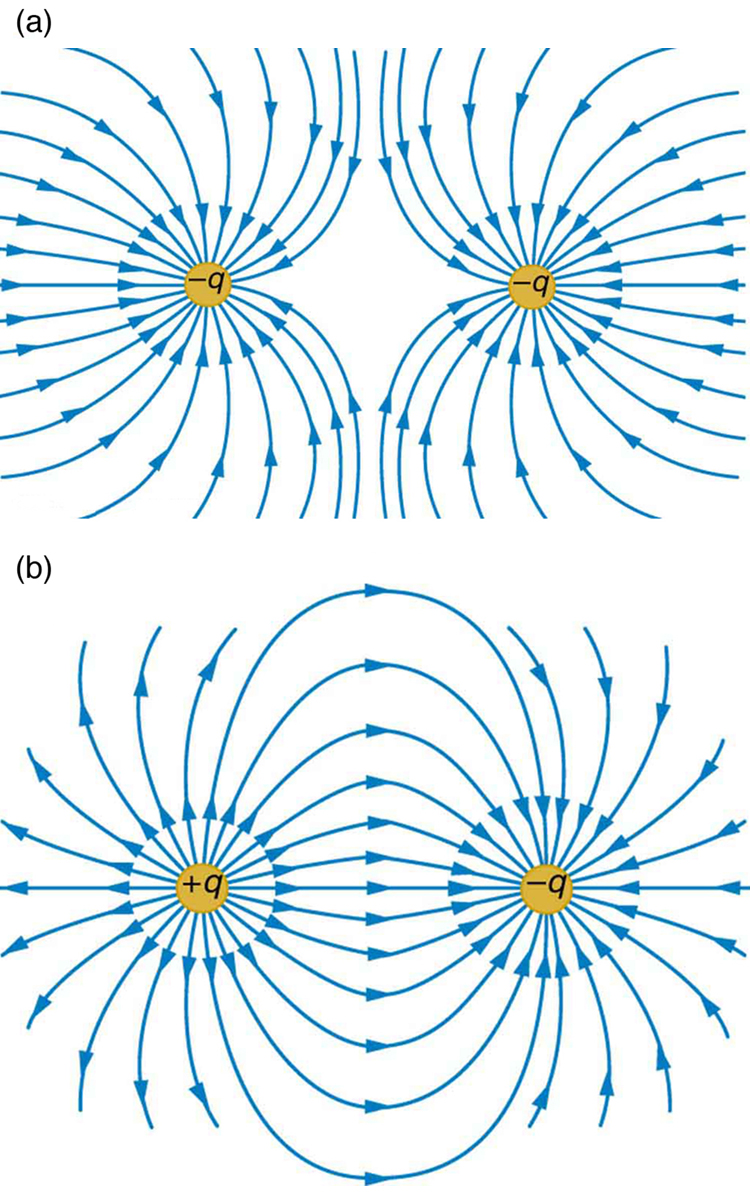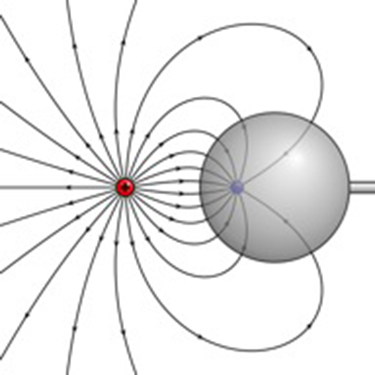7.4 Electric field lines  (Page 2/6)

 Page 2 / 6

[link] (b) shows the electric field of two unlike charges. The field is stronger between the charges. In that region, the fields from each charge are in the same direction, and so their strengths add. The field of two unlike charges is weak at large distances, because the fields of the individual charges are in opposite directions and so their strengths subtract. At very large distances, the field of two unlike charges looks like that of a smaller single charge.Two positive point charges q 1 size 12{q rSub { size 8{1} } } {} and q 2 size 12{q rSub { size 8{2} } } {} produce the resultant electric field shown. The field is calculated at representative points and then smooth field lines drawn following the rules outlined in the text.(a) Two negative charges produce the fields shown. It is very similar to the field produced by two positive charges, except that the directions are reversed. The field is clearly weaker between the charges. The individual forces on a test charge in that region are in opposite directions. (b) Two opposite charges produce the field shown, which is stronger in the region between the charges.

We use electric field lines to visualize and analyze electric fields (the lines are a pictorial tool, not a physical entity in themselves). The properties of electric field lines for any charge distribution can be summarized as follows:

1. Field lines must begin on positive charges and terminate on negative charges, or at infinity in the hypothetical case of isolated charges.
2. The number of field lines leaving a positive charge or entering a negative charge is proportional to the magnitude of the charge.
3. The strength of the field is proportional to the closeness of the field lines—more precisely, it is proportional to the number of lines per unit area perpendicular to the lines.
4. The direction of the electric field is tangent to the field line at any point in space.
5. Field lines can never cross.

The last property means that the field is unique at any point. The field line represents the direction of the field; so if they crossed, the field would have two directions at that location (an impossibility if the field is unique).

Section summary

• Drawings of electric field lines are useful visual tools. The properties of electric field lines for any charge distribution are that:
• Field lines must begin on positive charges and terminate on negative charges, or at infinity in the hypothetical case of isolated charges.
• The number of field lines leaving a positive charge or entering a negative charge is proportional to the magnitude of the charge.
• The strength of the field is proportional to the closeness of the field lines—more precisely, it is proportional to the number of lines per unit area perpendicular to the lines.
• The direction of the electric field is tangent to the field line at any point in space.
• Field lines can never cross.

Conceptual questions

Compare and contrast the Coulomb force field and the electric field. To do this, make a list of five properties for the Coulomb force field analogous to the five properties listed for electric field lines. Compare each item in your list of Coulomb force field properties with those of the electric field—are they the same or different? (For example, electric field lines cannot cross. Is the same true for Coulomb field lines?)

Problem exercises

(a) Sketch the electric field lines near a point charge $+q$ . (b) Do the same for a point charge $–3.00q$ .

Sketch the electric field lines a long distance from the charge distributions shown in [link] (a) and (b)

[link] shows the electric field lines near two charges ${q}_{1}$ and ${q}_{2}$ . What is the ratio of their magnitudes? (b) Sketch the electric field lines a long distance from the charges shown in the figure.The electric field near two charges.

Sketch the electric field lines in the vicinity of two opposite charges, where the negative charge is three times greater in magnitude than the positive. (See [link] for a similar situation).

what is math number
x-2y+3z=-3 2x-y+z=7 -x+3y-z=6
Need help solving this problem (2/7)^-2
x+2y-z=7
Sidiki
what is the coefficient of -4×
-1
Shedrak
the operation * is x * y =x + y/ 1+(x × y) show if the operation is commutative if x × y is not equal to -1
An investment account was opened with an initial deposit of $9,600 and earns 7.4% interest, compounded continuously. How much will the account be worth after 15 years? Kala Reply lim x to infinity e^1-e^-1/log(1+x) given eccentricity and a point find the equiation Moses Reply 12, 17, 22.... 25th term Alexandra Reply 12, 17, 22.... 25th term Akash College algebra is really hard? Shirleen Reply Absolutely, for me. My problems with math started in First grade...involving a nun Sister Anastasia, bad vision, talking & getting expelled from Catholic school. When it comes to math I just can't focus and all I can hear is our family silverware banging and clanging on the pink Formica table. Carole I'm 13 and I understand it great AJ I am 1 year old but I can do it! 1+1=2 proof very hard for me though. Atone hi Adu Not really they are just easy concepts which can be understood if you have great basics. I am 14 I understood them easily. Vedant find the 15th term of the geometric sequince whose first is 18 and last term of 387 Jerwin Reply I know this work salma The given of f(x=x-2. then what is the value of this f(3) 5f(x+1) virgelyn Reply hmm well what is the answer Abhi If f(x) = x-2 then, f(3) when 5f(x+1) 5((3-2)+1) 5(1+1) 5(2) 10 Augustine how do they get the third part x = (32)5/4 kinnecy Reply make 5/4 into a mixed number, make that a decimal, and then multiply 32 by the decimal 5/4 turns out to be AJ how Sheref can someone help me with some logarithmic and exponential equations. Jeffrey Reply sure. what is your question? ninjadapaul 20/(×-6^2) Salomon okay, so you have 6 raised to the power of 2. what is that part of your answer ninjadapaul I don't understand what the A with approx sign and the boxed x mean ninjadapaul it think it's written 20/(X-6)^2 so it's 20 divided by X-6 squared Salomon I'm not sure why it wrote it the other way Salomon I got X =-6 Salomon ok. so take the square root of both sides, now you have plus or minus the square root of 20= x-6 ninjadapaul oops. ignore that. ninjadapaul so you not have an equal sign anywhere in the original equation? ninjadapaul hmm Abhi is it a question of log Abhi 🤔. Abhi I rally confuse this number And equations too I need exactly help salma But this is not salma it's Faiza live in lousvile Ky I garbage this so I am going collage with JCTC that the of the collage thank you my friends salma Commplementary angles Idrissa Reply hello Sherica im all ears I need to learn Sherica right! what he said ⤴⤴⤴ Tamia hii Uday hi salma hi Ayuba Hello opoku hi Ali greetings from Iran Ali salut. from Algeria Bach hi Nharnhar A soccer field is a rectangle 130 meters wide and 110 meters long. The coach asks players to run from one corner to the other corner diagonally across. What is that distance, to the nearest tenths place. Kimberly Reply Jeannette has$5 and \$10 bills in her wallet. The number of fives is three more than six times the number of tens. Let t represent the number of tens. Write an expression for the number of fives.
What is the expressiin for seven less than four times the number of nickels
How do i figure this problem out.
how do you translate this in Algebraic Expressions
why surface tension is zero at critical temperature
Shanjida
I think if critical temperature denote high temperature then a liquid stats boils that time the water stats to evaporate so some moles of h2o to up and due to high temp the bonding break they have low density so it can be a reason
s.
Need to simplify the expresin. 3/7 (x+y)-1/7 (x-1)=
. After 3 months on a diet, Lisa had lost 12% of her original weight. She lost 21 pounds. What was Lisa's original weight?
Got questions? Join the online conversation and get instant answers!By Jordon HumphreysByByByBy